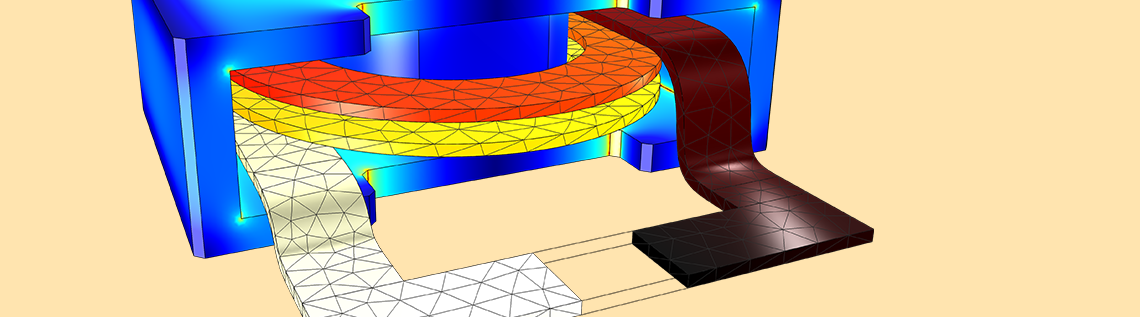# 如何使用 COMSOL 进行电热分析？

2020年 1月 13日

### 电磁损耗的热源计算

#### 焦耳热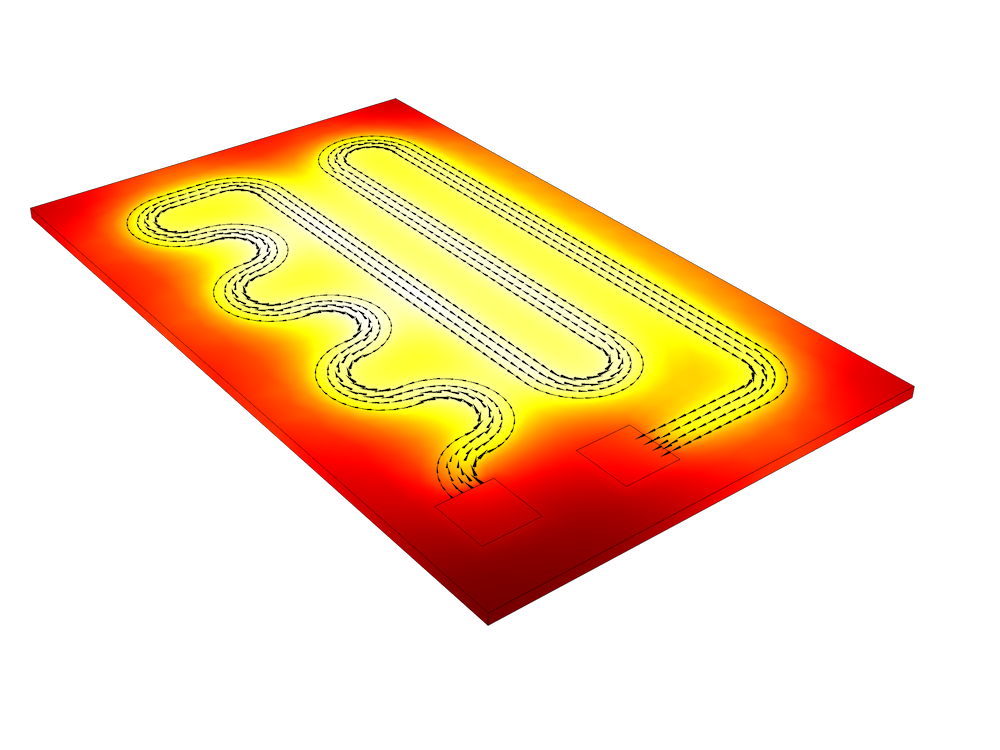Q_{e}=Q_{rh}添加为热源添，在频域中，Q_{rh}=\frac{1}{2}Re(\bold{J}\cdot\bold{E^*})或在时域中，Q_{rh}=\bold{J}\cdot\bold{E}

\bold{J}=\sigma\bold{E}

\bold{D}=\epsilon_0\epsilon_r\bold{E}=\epsilon_0(\epsilon_r’-j\epsilon_r”)\bold{E}

#### 感应加热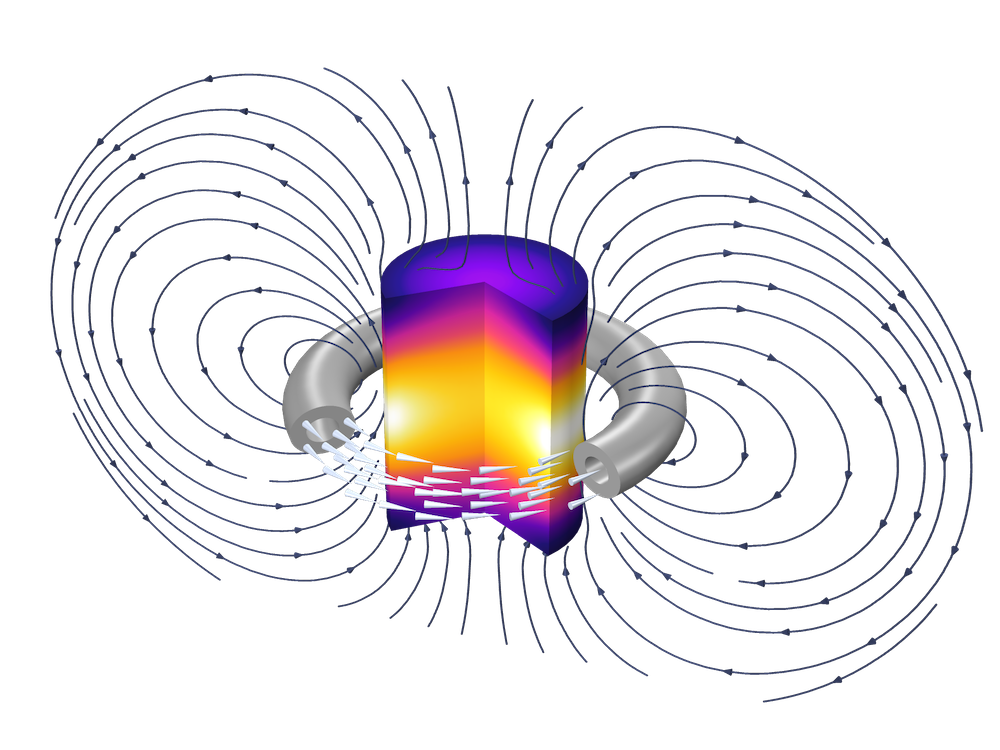Q_{e}=Q_{rh}+Q_{ml}添加为热源项,在频域中，Q_{rh}=\frac{1}{2}Re(\bold{J}\cdot\bold{E^*})Q_{ml}=\frac{1} {2}Re(i\omega\bold{B}\cdot\bold{H^*});在时域中，Q_{rh}=\bold{J}\cdot\bold{E}，而 Qml 与磁滞模型有关。

\bold{J}=\sigma\bold{E}

\bold{B}=\mu_0\mu_r\bold{H}=\mu_0(\mu_r’-j\mu_r”)\bold{H}

#### 微波加热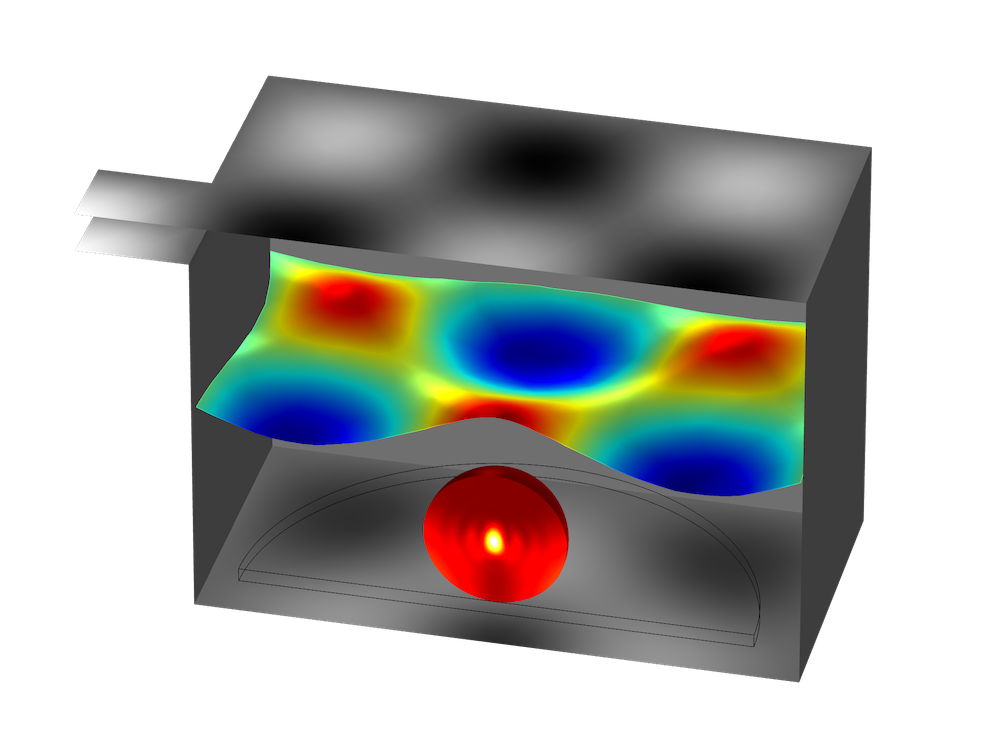Q_{e}=Q_{rh}+Q_{ml} 添加为热源项，在频域中，Q_{rh}=\frac{1}{2}Re(\bold{J}\cdot\bold{E^*}) ， Q_{ml}=\frac{1}{2}Re(i\omega\bold{B}\cdot\bold{H^*})。如上图所示，在频域中，用电导率（σ），复磁导率（µ”）和复相对介电常数（ε”）表示材料损耗。

#### 激光加热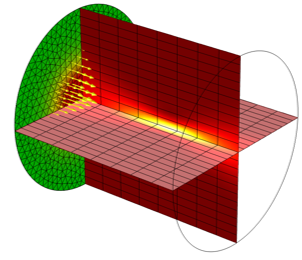Q_{e}=Q_{rh}+Q_{ml} 添加为热源项，在频域中，Q_{rh}=\frac{1}{2}Re(\bold{J}\cdot\bold{E^*})Q_{ml}=\frac{1} {2}Re(i\omega\bold{B}\cdot\bold{H^*})。如上图所示，在频域中，用电导率（σ），复磁导率（µ”）和复相对介电常数（ε”）表示损耗的材料特性。

1. 频域-稳态
2. 频域-瞬态
3. 频域-稳态，单向耦合
4. 频域-瞬态，单向耦合

1. 导热系数
2. 损耗角正切，损耗角

### 下一步

#### 评论 (11)

##### 留言##### 老师 任
2021-07-16##### Qihang Lin
2021-08-12 COMSOL 员工##### 老师 任
2021-08-20##### Qihang Lin
2021-08-23 COMSOL 员工##### 以 欣
2021-08-26##### Qihang Lin
2021-08-26 COMSOL 员工##### Cheng Dai
2021-08-24##### Qihang Lin
2021-08-26 COMSOL 员工##### 万伦 徐
2021-10-04##### hao huang
2021-10-13 COMSOL 员工